Function Repository Resource:

# AstroAngularDistance

Compute the angular distance between astronomical entities

Contributed by: Jeff Bryant
 ResourceFunction["AstroAngularDistance"][entity1,entity2] computes the current angular distance between entity1 and entity2. ResourceFunction["AstroAngularDistance"][entity1,entity2,date] computes the angular distance between entity1 and entity2 on the specified date. ResourceFunction["AstroAngularDistance"][entity1,entity2,{date1,date2,…,daten}] computes the angular distance between entity1 and entity2 on multiple dates.

## Details and Options

Valid domains for entities or entity classes can be from any of "Planet", "PlanetaryMoon", ”MinorPlanet", ”Comet", ”Exoplanet", ”Star", ”StarCluster", ”Nebula", ”Galaxy", ”Supernova", ”Pulsar" or ”AstronomicalRadioSource".
date must be a DateObject.
Shorter dates can be used: DateObject[{y}] is equivalent to DateObject[{y,1,1,0,0,0}], DateObject[{y,m}] to DateObject[{y,m,1,0,0,0}], etc.
For a list of dates, the result is returned as a TimeSeries.
Angular distances are returned in angular degrees by default.
Entities found in the solar solar system return results that change with time.
Angular distances to extra‐solar system objects are computed for a fixed epoch and ignore effects such as proper motion and precession.

## Examples

### Basic Examples (3)

Compute the current angular distance between two objects:

 In:=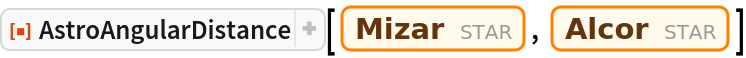Out=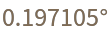In:=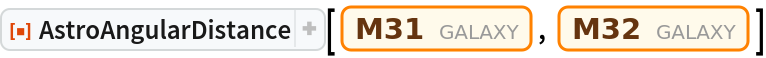Out=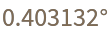Compute the angular distance between two objects on a specified date:

 In:=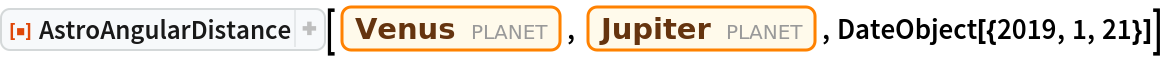Out=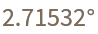Compute the angular distance between two objects over a range of dates:

 In:=Out=In:=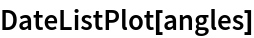Out=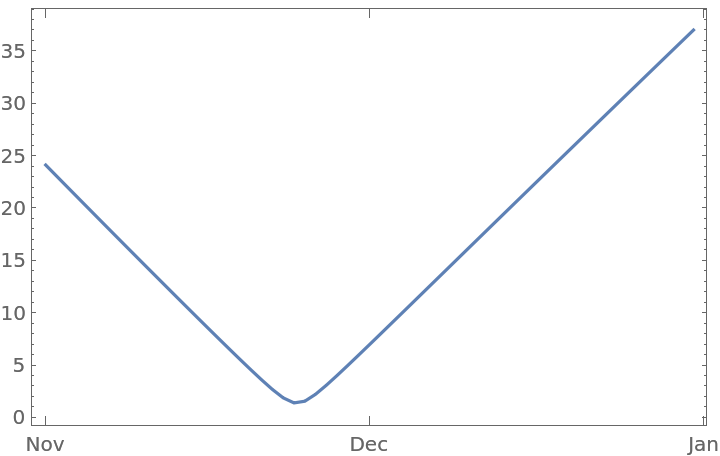### Scope (1)

Entities can be in different domains:

 In:=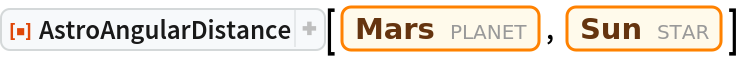Out=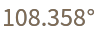### Options (1)

The units returned by AstroAngularDistance can be chosen using the option TargetUnits:

 In:=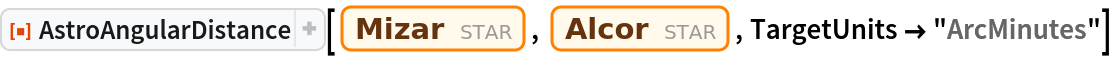Out=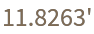### Possible Issues (1)

Since angles are measures as viewed from Earth, Earth itself cannot be included as one of the entities:

 In:=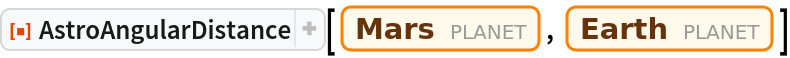Out=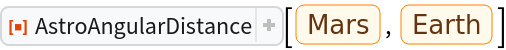## Version History

• 2.0.0 – 06 January 2021
• 1.0.0 – 25 July 2019